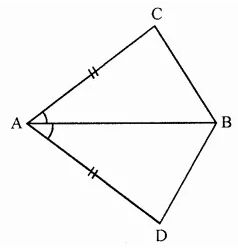# ML Aggarwal Class 7 Solutions for ICSE Maths Chapter 12 Congruence of Triangles Ex 12.1

## ML Aggarwal Class 7 Solutions for ICSE Maths Chapter 12 Congruence of Triangles Ex 12.1

Question 1.
If ΔABC and ΔDEF are congruent under the correspondence ABC ↔ FED, write all the corresponding congruent parts of the triangles.
Solution:Question 2.
If ΔDEF = ΔBCA, then write the part(s) of ΔBCA that correspond to
(i) ∠E
(ii) $$\bar { EF }$$
(iii) ∠F
(iv) $$\bar { DF }$$
Solution:Question 3.
In the figure given below, the lengths of the sides of the triangles are indicated. By using SSS congruency rule, state which pairs of triangles are congruent. In the case of congruent triangles, write the result in symbolic form: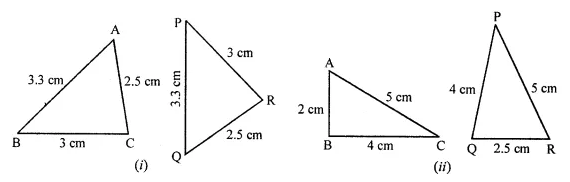Solution:Question 4.
In the given figure, AB = 5 cm, AC = 5 cm, BD = 2.5 cm and CD = 2.5 cm
(i) State the three pairs of equal parts in ΔADB and ΔADC
(iii) Is ∠B = ∠C? Why?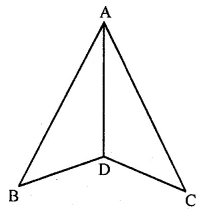Solution: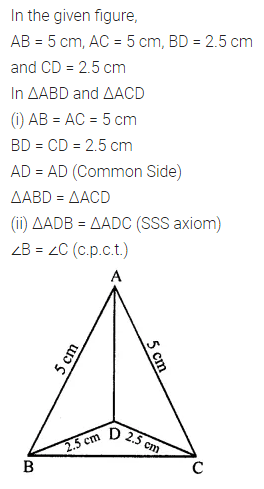Question 5.
In the given figure, AB = AC and D is the mid-point of $$\bar { BC }$$.
(i) State the three pairs of equal parts in ΔADB and ΔADC.
(iii) Is ∠B = ∠C? Why?Solution: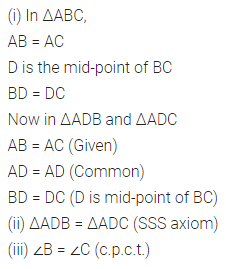Question 6.
In the figure given below, the measures of some parts of the triangles are indicated. By using SAS rule of congruency, state which pairs of triangles are congruent. In the case of congruent triangles, write the result in symbolic form.Solution: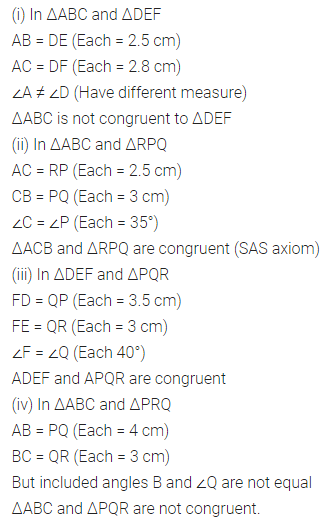Question 7.
By applying SAS congruence rule, you want to establish that ΔPQR = ΔFED. If is given that PQ = EF and RP = DF. What additional information is needed to establish the congruence?
Solution: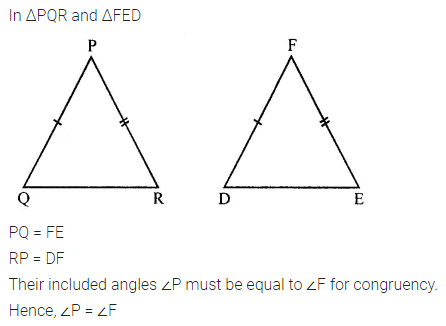Question 8.
You want to show that ΔART = ΔPEN(a) If you have to use SSS criterion, then you need to show
(i) AR = ……….
(ii) RT = ……….
(iii) AT = …………
(b) If it is given that ∠T = ∠N and you are to use the SAS criterion, you need to have
(i) RT = ………. and (ii) PN = ……….
Solution: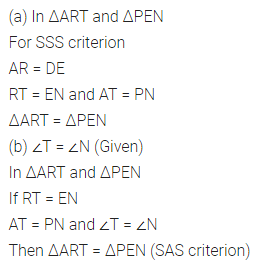Question 9.
You have to show that ΔAMP = ΔAMQ.
In the following proof, supply the missing reasons.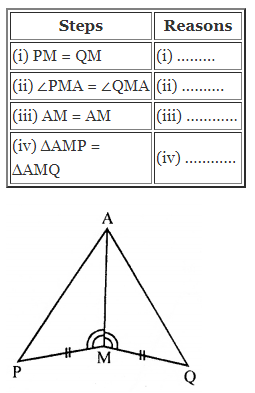Solution:Question 10.Solution:
In the given figure:
(i) State three pairs of equal parts in ΔPSR and ΔRQP.
(ii) Is ΔPSR = ΔRQP? Give reasons
(iii) Is PS = RQ? Why?
(iv) Is ∠S = ∠Q? Why?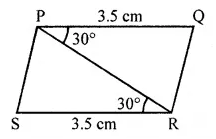Solution: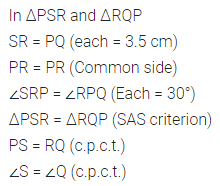Question 11.
In the given figure, AB = DC and ∠ABC = ∠DCB.
(i) State three pairs of equal parts in AABC and ADCB.
(ii) Is ΔABC = ΔDCB? Give reasons.
(iii) Is AC = DB? Why?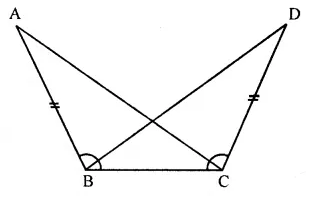Solution: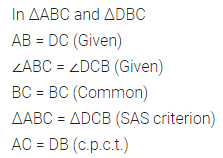Question 12.